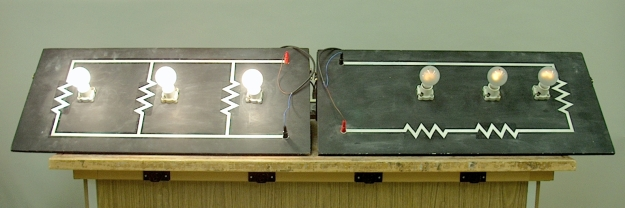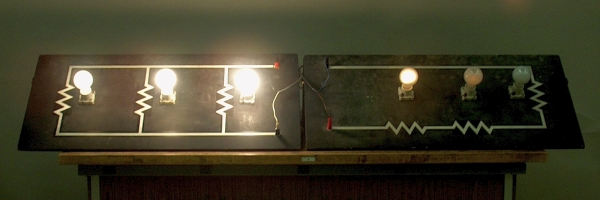OK. These are actually AC circuits. Since the loads are almost purely resistive, i.e., there are no capacitances or inductances (or they are small enough to be negligible), and since the rms (root-mean-square) AC voltage and current behave in purely resistive circuits as DC voltage and current do, the two circuits shown above are equivalent to the corresponding DC circuits. The AC from the wall is sinusoidal. The rms voltage for a sinusoid is 0.707Vp, where Vp is the peak voltage. Similarly, the rms current through a resistor is 0.707ip, where ip is the peak current. These effective values correspond to the DC values that would give the same power dissipation in the resistor. These are slightly different from the average voltage and current, which are 0.639Vp and 0.639ip for a sinusoid. For AC from the wall, the rms voltage is approximately 120 V, and the average voltage is about 110 V.

Each board has three 40-watt bulbs, connected as shown by the resistor circuit painted on it. The board on the left has the bulbs arranged, of course, in parallel, and the board on the right has them in series. Since power, P, equals iV, P/V = i, so at 120 V, a 40-watt bulb draws 1/3 A. (The units in iV are (C/s)(N-m/C), or J/s, which are watts.) For a given resistance, V = iR, so the bulb’s resistance (when it has 120 volts across it) is 120/(1/3), or 360 ohms. (We also know by the two equations above that P = i2R, which gives R as 40/(1/9), or 360 ohms.)

When the bulbs are connected in parallel, each bulb has 120 V across it, each draws 1/3 A, and each dissipates 40 watts. In this circuit, all bulbs glow at their full brightness. The total power dissipated in the circuit is three times 40, or 120 watts (or 3(1/3) A × 120 V = 120 W).

In the series circuit, any current that flows through one bulb must go through the other bulbs as well, so each bulb draws the same current. Since all three bulbs are 40-watt bulbs, they have the same resistance, so the voltage drop across each one is the same and equals one-third of the applied voltage, or 120/3 = 40 volts. The resistance of a light bulb filament changes with temperature, but if we ignore this, we can at least roughly estimate the current flow and power dissipation in the series circuit. We have 120 V/(360 + 360 + 360) ohms = 1/9 A. The power dissipated in each bulb is either (1/9)2 × 360 = 4.44 watts, or (1/9) × 40 = 4.44 watts. The total power dissipated in the circuit is three times this, or 13.3 watts ((1/9)2 × 3(360) = 1080/81 = 13.3 W, or (1/9) A × 120 V = 13.3 W).

With fresh light bulbs, direct measurement with an ammeter shows that the actual current flowing in the parallel circuit is 0.34 A for one bulb, 0.68 A for two bulbs and 1.02 A for three bulbs, and in the series circuit it is 0.196 A. So the current, and thus the dissipated power (23.5 watts), in the series circuit are almost twice what we arrived at above.

An “ohmic” resistance is one that stays constant regardless of the applied voltage (and thus also the current). If the light bulbs behaved this way, the measured current in the series circuit would agree with the estimate above. Even though they do not, this demonstration gives a good sense of the difference in behavior between a series and parallel circuit made with three identical resistors.

What happens if the light bulbs are not all of the same wattage rating?

An interesting variation of this demonstration is to show what happens when we put light bulbs of three different wattages in each circuit. A good choice is to keep one 40-W light bulb in each circuit, and then add a 60-W bulb and a 100-W bulb. In the parallel circuit, as noted above, the voltage across each bulb is the same (120 V), so each bulb draws the current that it would if it alone were connected to the wall, and the intensities of the bulbs thus vary as you would expect from the wattage ratings. The 100-W bulb is the brightest, the 40-W bulb is the dimmest, and the 60-W bulb is somewhere in between. When we put the same combination of bulbs in series, an interesting thing happens. Because both the 60-W bulb and the 100-W bulb have lower resistance than the 40-W bulb, the current through the circuit is somewhat higher than for the three 40-W light bulbs in series, and the 40-W bulb glows more brightly than it did when it was in series with two other 40-W bulbs. The current through this circuit measures 0.25 A. This is about 76% of the 0.33 A that the 40-W bulb would draw by itself, half the 0.5 A that the 60-W bulb would draw, and 30% of the 0.83 A that the 100-W bulb would draw. At this current, the 40-W bulb lights fairly brightly, the 60-W bulb just barely glows, and the 100-W bulb does not light at all. The photograph below shows the operation of these two circuits:The bulbs in each circuit, from left to right, are a 40-W, 60-W and a 100-W light bulb. In the parallel circuit, the bulbs obviously increase in brightness from left to right. In the series circuit, the brightness decreases from left to right. The measured voltages in the circuit are 120 V across all three bulbs, 109 V across the 40- and the 60-W bulbs, and 78 V across the 40-Watt bulb. The voltage drop across the 60-W bulb is thus 31 V, and it is 11 V across the 100-W bulb. Multiplying each of these by the 0.25-A current, we find that in the series circuit, the 40-W bulb dissipates about 20 watts, the 60-W bulb dissipates 7.8 watts, and the 100-W bulb dissipates about 2.8 watts, which corresponds with the relative intensities we observe for the three bulbs.

References:

1) Howard V. Malmstadt, Christie G. Enke and Stanley R. Crouch. Electronics and Instrumentation for Scientists (Menlo Park, California: The Benjamin/Cummings Publishing Company, Inc., 1981), pp. 31-32.